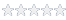# How to use Microsoft Excel to calculate a statistical/mathematical/financial…

#### Knowledge Base Article # Q200080

Summary Example to show how calculates a statistical/mathematical/financial or any other excel function from ATEasy.Login to rate article
The example creates a hidden Excel workbook, fill the data and calculate TINV value and return it to ATEasy.

TINV Excel function used in this example returns the t-value of the Student's t-distribution as a function of the probability and the degrees of freedom.

You can modify the example to calculate and to use any excel mathematical/statistical/financial formula.

ob: Object

ob=CreateObject("Excel.Application")
if ob=Nothing
MsgBox("Unable to Create Excel.Application. Check if MS Excel is intsalled properly. Aborting...")
abort
endif
ob.Caption="ATEasy Excel Function Call Using COM"
ob.Visible=TRUE     ! show/activate execl main window

ob.Cells.Item(1, 1).Value = 0.054644927
ob.Cells.Item(2, 1).Value = 60.0
ob.Cells.Item(1, 2).Value = "=TINV(A1, A2)"

print ob.Cells.Item(1, 2).Value

ob.Workbooks.Item(1).Close(FALSE)    ! close workbook do not save changes
ob.Quit()
ob=Nothing
Article Date 12/7/2006
Keywords ATEasy, Excel, CreateObject, TINV, Object

 Login to rate article>